# 6. Quality Concept¶

This chapter explains how MQC computes quality based on the imported data, derived measures (both are introduced in Dimensions and Structures) and a Quality Model. Additionally it describes how quality is structured in MQC.

## 6.1. Quality Computation¶

When data is collected and imported in MQC, it is handled in terms of measures. In the following, we consider one artifact and one revision.

A measurement – that is the set of operations executed to determine values for measures – is applied and it yields a collection of measures including their measure values (variables). Either these measures can be base measures coming directly from the data source or derived measures, i.e. computed from base measures or other derived measures (see Figure 5.10).

One, two or multiple of these base and derived measures are used to calculate a quality value between 0 and 1. This quality value is associated to a so-called quality property. Quality properties define the lowest level of computed quality. Examples of quality properties are “Guideline Compliance” or “Test Sequence Compliance”.

For each quality property, a measurement function defines how to calculate the quality value by using base and derived measures.

Note

Quality values always need to be between 0 and 1. This is ensured by MQC even if the measurement function yields a value outside these boundaries.

The workflow from measuring the data to calculating the quality value for a quality property is shown in Figure 6.1.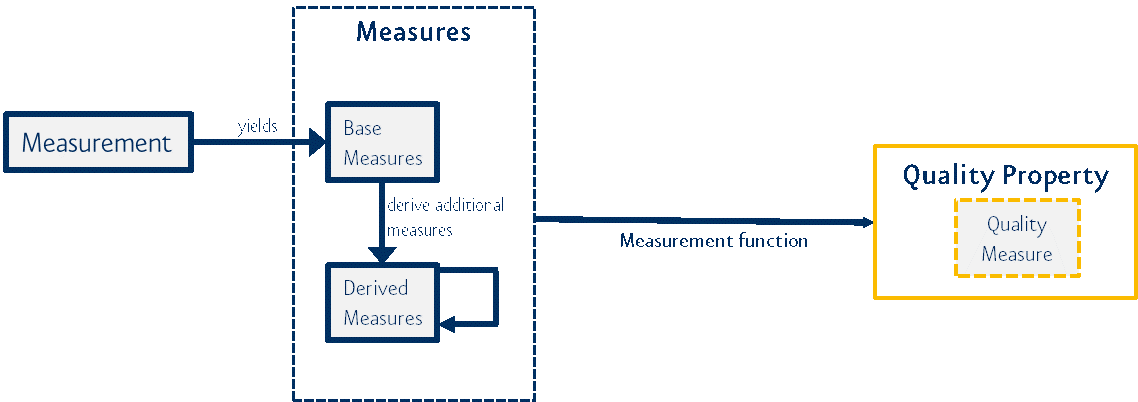Figure 6.1 Workflow from collecting data with a measurement to calculating a quality value by a measurement function

An example for a simple measurement function is the computation of a quality value for the quality property “Model Decision Coverage” using the base measure values `Reached` and `Total`:

[Model Decision Coverage.Reached] / [Model Decision Coverage.Total]

A more complex measurement function calculates the “Local Complexity” using the three different base measure variables `Good`, `Acceptable` and `Bad`:

1.0*[Local Complexity.Good] + 0.8*[Local Complexity.Acceptable] + 0.2*[Local Complexity.Bad] / [Local Complexity.Good] + [Local Complexity.Acceptable] + [Local Complexity.Bad]

The given base measures are weighted accordingly to compute a normalized quality value, i.e. between 0 and 1.

Please note that the quality value of a quality property can also be calculated by using the results of more than one measurement. In this case, the measurement function to compute the quality value is used for all the different measurements from the same data source. One example is the quality property “Guideline Compliance”, where for the measurements “Measuring MISRA compliance”, “Measuring MAAB compliance” and “Measuring TargetLink Known problems compliance”, three quality values based on the same measurement function are computed, see Figure 6.2.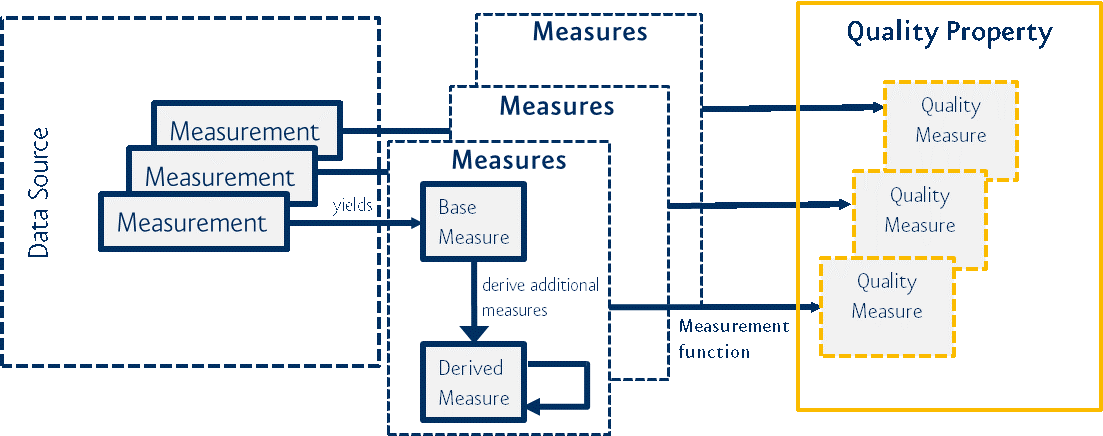Figure 6.2 Measurements from the same data source use the same measurement function for computing the quality values

Please refer to Quality Properties for details on how to configure quality properties and measurement functions in MQC.

## 6.2. Quality Bins¶

Quality values can be mapped to quality bins. A quality bin is a kind of category that contains a set of quality values of a certain value range. Per default, MQC uses a quality bin definition as follows:

• Bad, for quality values in [0%, 20%]

• Acceptable, for quality values in ]20%, 80%]

• Good, for quality values in ]80%, 100%].

Note

MQC allows to customize these categories. It is possible to configure less or more bins than MQC provides as default, to adapt the value ranges of the bins and even to choose an alternative color scheme. For more details see Quality Model and Quality Bin Configuration.

Next to the quality value, the quality bin adds an additional attribute to each quality property (see Figure 6.3).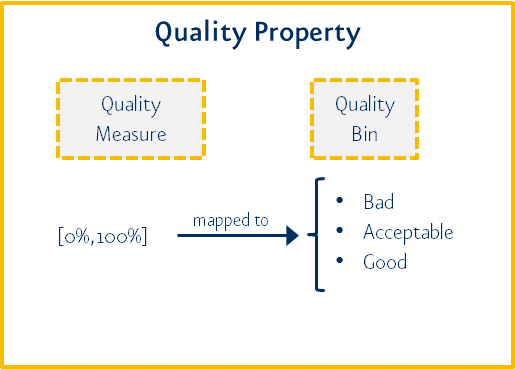Figure 6.3 Quality bin as additional attribute in quality property

By counting the number of elements in the bin categories, e.g. in the categories “Good”, “Acceptable” and “Bad”, MQC is able to provide a quality value distribution.

## 6.3. Quality Model Structure¶

Quality properties are the computable atomic elements of the quality model with values between 0 and 1. By an adjustable aggregation method, an overall quality value is calculated.

MQC allows the user to implement a general quality model that complies with ISO 25010. The user can define quality properties and a quality structure according to the project and process needs. Figure 6.4 shows an example structure with two levels, “Characteristics” and “Subcharacteristics”, of a quality model.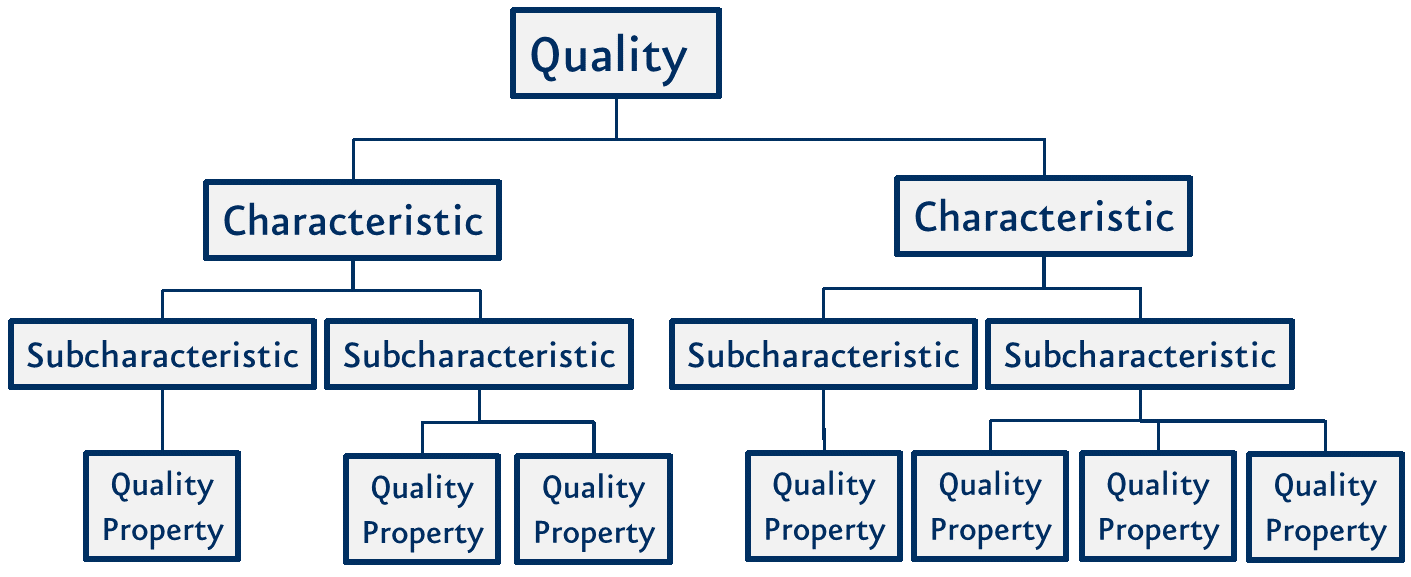Figure 6.4 Quality Model – Concept (ISO 25010, p. 2)

## 6.4. Quality Aggregation¶

Looking at the three dimensions ‘Measure’, ‘Artifact’ and ‘Revision’ all the quality values build a quality cube, see Figure 6.5. Each quality property represents a slice.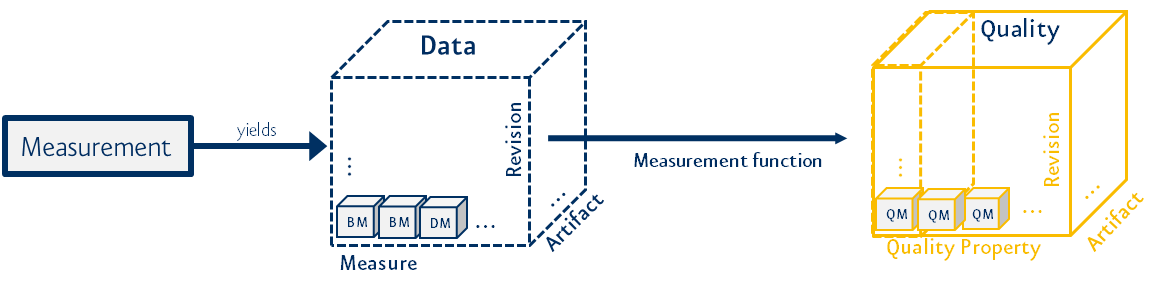Figure 6.5 From data cube to quality cube

Based on the quality value for each quality property per revision and artifact, a first aggregation step is performed. For every single quality property, the corresponding quality values for all artifacts are aggregated by calculating the average (see Figure 6.6).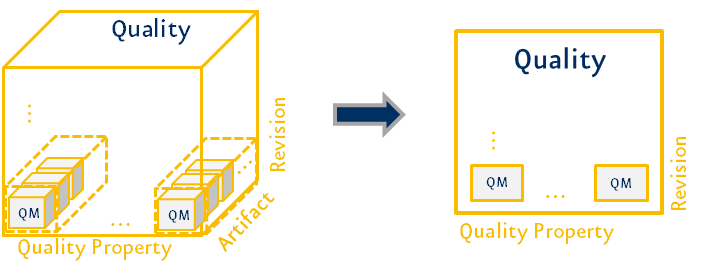Figure 6.6 First aggregation step with respect to all artifacts

This is the basis for further aggregation in the quality structure.

According to the Quality Model defined by the user, the quality properties are used for the quality aggregation into their parent structure elements and the overall quality. Each of these aggregations is done using the associated quality properties directly as a base. There is no technical hierarchy in the quality aggregation calculation.

All aggregations currently use the (weighted) average calculation as aggregation function, e.g. the average of all quality property measures for a certain structure-element is calculated to gain the quality value for this particular structure-element and so on.

Note

The structure does not influence the overall quality. It can define different structure levels and elements to group and better visualize quality properties, especially for large projects.